Electron. J. Diff. Eqns., Vol. 2006(2006), No. 15, pp. 1-9.

### A Liouville theorem for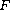-harmonic maps with finite-energy M'hamed Kassi

Abstract:
Let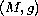be a-dimensional complete Riemannian manifold with a pole, and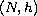a Riemannian manifold. Let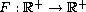be a strictly increasing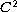function such that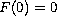and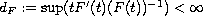. We show that if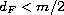, then every-harmonic map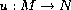with finite-energy (i.e a local extremal of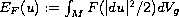and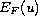is finite) is a constant map provided that the radial curvature ofsatisfies a pinching condition depending to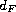.

Submitted March 24, 2005. Published January 31, 2006.
Math Subject Classifications: 58E20, 53C21, 58J05.
Key Words: F-harmonic maps; Liouville propriety; Stokes formula; comparison theorem.

Show me the PDF file (212K), TEX file, and other files for this article.M'hamed Kassi Equipe d'Analyse Complexe, Laboratoire d'Analyse Fonctionnelle Harmonique et Complexe Département de Mathématiques, Faculté des Sciences Université Ibn Tofail, Kénitra, Maroc email: mhamedkassi@yahoo.fr